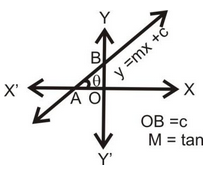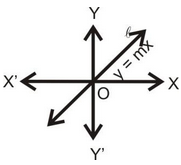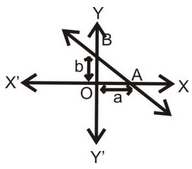# DIFFERENCE FORMS OF LINE

## SLOPE OF A LINE:

If a line makes an angle θ with positive direction of x-axis then tangent of this angle is called the slope of a line, it is denoted by m i.e. m = tanθ.

(i) Slope - intercept from is y = mx + c where m is the slope of line and c is intercept made by line with Y-axis.(ii) The equation of a line passing through origin is y = mx. Here c= 0 then the line passes always from origin.(iii) Intercept from of line is x/a + y/b = 1 where a & b are intercepts on positive direction of x-axis and y-axis respectively made by line.